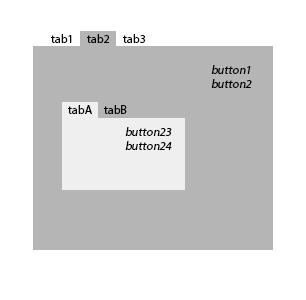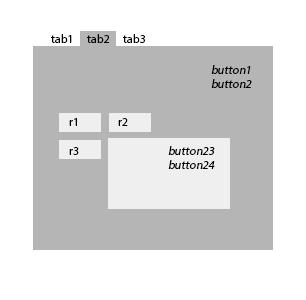#### Howdy, Stranger!

We are about to switch to a new forum software. Until then we have removed the registration on this forum.

# [ControlP5] tab in tab (possible?)

edited August 2014

hello again, =:)
is it possible to make a tab in another tab?
f.e.Tagged:

• No, but take a look at the ControlP5 Accordion example, perhaps it is something you can use if you comment out two lines and add a line like this:

``````  // accordion.open(0,1,2);
// accordion.setCollapseMode(Accordion.MULTI);
accordion.open(0);
``````
• yes, it works really great, but the "tabs" from the accordion should be always on the top.is there any option to make it like this? what should i do for it?

• Perhaps you could put them in Groups instead of in an accordion. Place all the Groups in the same location with the same dimensions. Then set the visibility to cycle through the different groups. Here is a proof-of-concept sketch that cycles through two groups by pressing the SPACE bar. This concept can of course be used for more groups and cycling could be triggered by anything (for example other buttons).

Proof-Of-Concept

``````import controlP5.*;
ControlP5 cp5;

int visibleGroup;
int numGroups = 2;

void setup() {
size(700, 400);
cp5 = new ControlP5(this);

// GROUP 1
.setPosition(300,100)
.setWidth(300)
.setBackgroundHeight(100)
.setBackgroundColor(color(255,50))
;

.setPosition(10,20)
.setSize(80,20)
.setGroup(g1)
;

.setPosition(10,60)
.setSize(80,20)
.setGroup(g1)
;

// GROUP 2
.setPosition(300,100)
.setWidth(300)
.activateEvent(true)
.setBackgroundColor(color(255,80))
.setBackgroundHeight(100)
.setLabel("Hello World.")
;

.setPosition(80,10)
.setSize(180,9)
.setGroup(g2)
;

.setPosition(80,20)
.setSize(180,9)
.setGroup(g2)
;

.setPosition(10,10)
.setSize(20,9)
.setGroup(g2)
;

// set initial visibility
setVisibleGroup(visibleGroup);
}

void draw() {
background(0);
}

void setVisibleGroup(int selected) {
for (int i=0; i<numGroups; i++) {
cp5.getGroup("g"+i).setVisible(i==selected);
}
}

void keyPressed() {
if (key == ' ') {
setVisibleGroup(++visibleGroup % numGroups);
}
}
``````
• ``````...up("g"+i).setVisible(i==selected)
++visibleGroup % numGroups
``````

very clever! ^:)^ (it took me quiet some time to understand how it works)

but this are my requirements:
- use the navi per buttons (f.e. radioButtons or Tabs(wich are on the top))
- don't see the background from the groups
- don't see the label-bar
- only one can be open (yes it works)

1. what would you prefer, make it with groups or otherwise?
2. and would you use with this requirements accordion additional?

Just try some things and look at the javadocs. For example to not see a background in the groups you can just remove the line .setBackgroundColor() and to remove the bar you can just add the line .hideBar() etc.

• ``````void setVisibleGroup(int selected) {
for (int i=0; i<numGroups; i++) {
cp5.getGroup("g"+i).setVisible(i==selected);
}
}
``````

Indeed very clever! My approach was always to make a function to set everything `false`.
And only then make the selected `true`! 8-|
However, I still think my simpler approach is slightly faster! :P

• thanks for your help. i could make it. and now it works perfectly (*)
here is my code:

``````import controlP5.*;
ControlP5 cp5;

void setup() {
size(400, 300);

cp5 = new ControlP5(this);

.setPosition(100, 100)
.hideBar() //is not necessary, if the Y-Position == 0 (cause then the bar disappears above
;          //                                          the window)

.setNoneSelectedAllowed(false)
;
r.activate(1);

.setPosition(50, 50)
.setGroup(g1)
;
.setPosition(width/2, height/2)
.setGroup(g3)
;
.setPosition(80, 10)
.setGroup(g2)
;
}

void draw() {
background(100);
}

void controlEvent(ControlEvent theEvent) {
if (theEvent.isFrom(r)) {
for (int i=1; i<=r.getArrayValue ().length; i++) {
cp5.getGroup("g"+i).setVisible(i==r.getValue());
}
}
}
``````
• Great! :-)